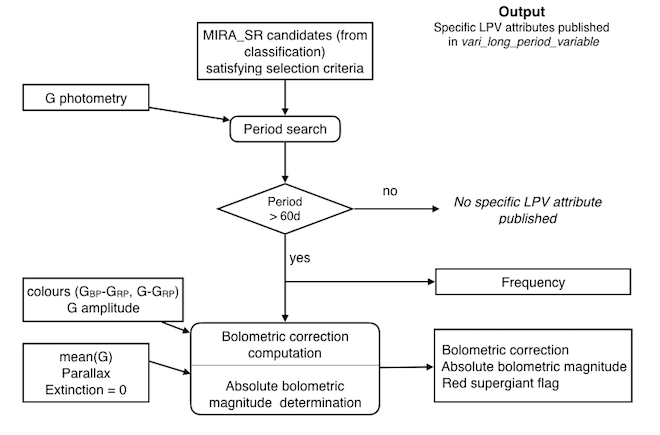# 7.7.4 Processing steps

The SOS LPV processing applied the following steps to the pre-selected LPVs as shown in Figure 7.5:Figure 7.5: Gaia DR2 Long Period Variable processing overview.
• Computation of a variability period based on the $G$ time series.
The period search method used is Least Square, applied to a frequency range from 0.001 c/d to 0.1 c/d with a frequency step of $5*10^{-5}$ c/d. In Gaia DR2, only sources with periods greater than 60 days are published, in particular because of the aliasing in the period search.

• Computation of bolometric correction.
As described in Section 7.7.3.

• Determination of the absolute bolometric magnitude M${}_{bol}$.
Using the Gaia parallax measurement (which was still preliminary at the time of our processing), the mean $G$-band magnitude, and the bolometric correction, the absolute bolometric magnitude was calculated using (with $\varpi$ in arcsec):

 $M_{bol}=m_{G}+\mathrm{BC}_{G}-A_{G}+5\log\varpi+5$ (7.14)

where $m_{G}$ is the mean $G$-band magnitude, $\mathrm{BC}_{G}$ the bolometric correction, $A_{G}$ the interstellar extinction which has been forced to 0 as it was not available as input and $\varpi$ the parallax given in arcseconds. The uncertainty of the absolute bolometric magnitude is derived via:

 $\sigma_{M_{bol}}=\sqrt{\sigma_{m_{G}}^{2}+\sigma_{BC}^{2}+\sigma_{A_{G}}^{2}+4% .715\,\varpi^{-2}\,\sigma_{\varpi}^{2}}$ (7.15)

Note that the uncertainty on the extinction has been forced arbitrarily to 0.05 mag. We point out that the absolute bolometric magnitude and its error is depending on the calculation type of the mean $G$ magnitude and its error. Due to the variability of LPVs the values of $m_{G}$ and $\sigma_{m_{G}}$ could be misleading. The derived light amplitudes in the $G$ band could also be underestimated compared to the real ones depending on the coverage and gaps of data points and hence on the quality of the lightcurve.

• Set of red supergiant flag.
Stars brighter than $M_{bol_{P}}$ (cf. (7.12)) are flagged as red supergiant.

Finally, each LPV candidate is published in the vari_long_period_variable table (Section 14.3.5) with the following attributes:

• one frequency (and the associated uncertainty),

• the bolometric correction (and the associated uncertainty),

• the absolute bolometric magnitude (and the associated uncertainty),

• a red super giant flag.